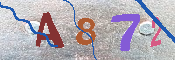## 细胞起源的时间——关于人和宇宙的设想（8）

1，问题引出

2，初始生命短段时期的生长繁殖活动

V=(4/3)π((nr)^3)

M=V/((4/3)π((100r)^3))=(n/100)^3

df/dt=(df/dh)(dh/dt)

df/dh=c

c为一常数，从而

df/dt=c(dh/dt)

f(h(t+T))=f(h(t))

df(t+T)/dt=c(dh(t+T)/dt)=c(dh(t+T)/d(t+T))

=c(dh(t)/dt)

3，有关细胞的计算与估计

1) 到10^4，即1万个小分子对时，

(10^4)/100=10^2，

M=(10^2)^3=10^6，

2) 到(10^5)/2，即5万个小分子对时，

((10^5)/2)/100=(10^3)/2，

M=((10^3)/2)^3=(10^9)/8=1.25×10^8，

3) 到(10^5)/(4/3)，即7万个小分子对时，

((10^5)/(4/3))/100=(10^3)/(4/3)，

M=((10^3)/(4/3))^3=(10^9)/(4/3)^3

=4.2×10^8，

0.34nmx(10^5)/(4/3)=25.5µm(微米)

4) 到10^5，即10万个小分子对时，

(10^5)/100=10^3，

M=(10^3)^3=10^9，

0.34nmx100000=34µm(微米)，

5) 到(10^5)/(3/4)，即13万个小分子对时，

((10^5)/(3/4))/100=(10^3)/(3/4)，

M=((10^3)/(3/4))^3=(10^9)/(3/4)^3

=20×10^8，

0.34nmx(10^5)/(3/4)=45.3µm(微米)，

4，生命起源与细胞起源的时间关系

［1］黎日工，细胞起源的草图——关于人和宇宙的设想（5）*